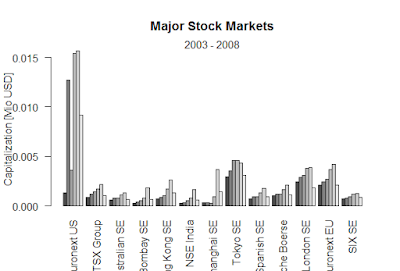Showing posts with label mathematical- statistical- probability and character functions-R. Show all posts
Showing posts with label mathematical- statistical- probability and character functions-R. Show all posts

## Mathematical and character functions-R

Absolute value
> abs(-4)
 4
Square root
> sqrt(25)
 5
Smallest integer not less than x
> ceiling(3.475)
 4
Largest integer not greater than x
> floor(3.475)
 3
Integer formed by truncating values in x toward 0
> trunc(5.99)
 5
> round(3.475, digits=2)
 3.48
Round x to the specified number of significant digits
> signif(3.475, digits=2)
 3.5
Cosine, sine, and tangent
> cos(2)
 -0.4161468
Arc-cosine, arc-sine, and arc-tangent
> acos(-0.416)
 1.999839
Hyperbolic cosine, sine, and tangent
> sinh(2)
 3.62686
Hyperbolic arc-cosine, arc-sine, and arc-tangent
> asinh(3.627)
 2.000037
Logarithm of x to the base n for convenience
log(x) is the natural logarithm
> log10(x) is the common logarithm.
> log(10)
 2.302585
> log10(10)
 1
Exponential function
> exp(2.3026)
 10.00015

### Black-Scholes formula-R

Black-Scholes formula-R > BlackScholes <- function(TypeFlag = c("c", "p"), S, X, Time, r, b, sigma) { TypeFla...